# MP Board Class 12th Chemistry Important Questions Chapter 4 Chemical Kinetics

## MP Board Class 12th Chemistry Important Questions Chapter 4 Chemical Kinetics

### Chemical Kinetics Important Questions

Chemical Kinetics Objective Type Questions

Question 1.

Question 1.
For most of the reactions, the value of temperature coefficient lies in between :
(a) 1 and 3
(b) 2 and 3
(c) 1 and 4
(d) 2 and 4.
(b) 2 and 3

Question 2.
For First order reaction value of tm is : (MP 2012 Supp.)
(a) $$\frac { 0.693 }{ { k }_{ 1 } }$$
(b) $$\frac { 2.303 }{ { k }_{ 1 } }$$
(c) $$\frac { 0.303 }{ { k }_{ 1 } }$$
(d) $$\frac {0.693}{t}$$
(a) $$\frac { 0.693 }{ { k }_{ 1 } }$$

Question 3.
A first order reaction gets completed to 75% in 32 minutes. How much time would have been required for 50% completion :
(a) 24 minute
(b) 16 minute
(c) 8 minute
(d) 4 minute.
(b) 16 minute

Question 4.
Hydrolysis of sucrose to glucose and fructose is :an example of:
(a) First order reaction
(b) Second order reaction
(c) Third order reaction
(d) Zero order reaction.
(a) First order reaction

Question 5.
Plants prepare starch in the process of:
(a) Flash photolysis
(b) Photolysis
(c) Photosynthesis
(d) None of these.
(c) PhotosynthesisQuestion 6.
For first order reaction the specific reaction constant depends upon :
(a) Concentration of reactants
(b) Concentration of products
(c) Time
(d) Temperature.
(d) Temperature.

Question 7.
In the reaction between A and B to form C, A represents first order and B represents second order. Rate equation will be written as :
(a) Rate = k [A]2[B]
(b) Rate = k [A] [B]2
(c) Rate = k [A]1/2[B]
(d) Rate = k [A] [B]1/2
(b) Rate = k [A] [B]2

Question 8.
The rate of chemical reaction depends upon : (MP 2017)
(a) Active mass
(b) Atomic mass
(c) Equivalent weight
(d) Molecular mass.
(a) Active mass

Question 9.
Arrhenius equation is :
(a) k = e-EaRT
(b) k = $$\frac { { E }_{ a } }{ RT }$$
(c) k = log$$\frac { { E }_{ a } }{ RT }$$
(d) k = Ae-Ea/RT
(d) k = Ae-Ea/RT

Question 10.
Unit of velocity constant of first order reaction :
(a) mol litre-1 sec-1
(b) mol-1 litre+1 sec-1
(c) sec-1
(d) mol litre-1 sec
(c) sec-1

Question 11.
Unit of velocity constant of zero order reaction :
(a) mol-1 litre-1 sec-1
(b) mol-1 litre+1 sec-1
(c) sec-1
(d) mol litre-1 sec
(a) mol-1 litre-1 sec-1Question 12.
Rate constant of a reaction increased with the increase of which of the following factor as :
(a) Pressure
(b) Temperature
(c) Concentration of reaction
(d) All of these.
(b) Temperature

Question 13.
Factor on which the rate constant of the 1st order reaction does not depend :
(a) Temperature
(b) Catalyst
(c) Activation energy
(d) Concentration of reactant.
(d) Concentration of reactant.

Question 14.
Minimum energy required for molecules to react is called :
(a) Potential energy
(b) Kinetic energy
(c) Nuclear energy
(d) Activation energy.
(d) Activation energy.

Question 15.
Law of mass action prepared by :
(a) Dalton
(b) Guddberg and Waaje
(c) Hunds and Mulliken
(d) Arrhenius.
(b) Guddberg and Waaje

Question 16.
Unit of reaction rate is:
(a) mol litre-1 sec-1
(b) mol-1 litre sec-1
(c) mol-1 litre-1 sec
(d) mol litre sec
(a) mol litre-1 sec-1

Question 2.
Fill in the blanks :

1. The rate of a reaction does not depends on the concentration of the reacting species, then the reaction is of ……………………
2. Half life period of a radioactive element is 140 days. On taking 1 gm element initially the amount left after 560 days will be ……………………
3. Fast reactions are completed in less than …………………… seconds.
4. Unit of rate constant for third order reaction is ……………………
5. Difference in the minimum and maximum energy state of reactants is called ……………………
6. Reactions which take place by the absorption of radiations are called …………………… (MP 2016)
7. The total number of molecules which participate in a reaction is called ……………………
8. In the mechanism of a reaction, the slowest step is called ……………………

1. Zero
2. $$\frac {1}{2}$$ gm
3. 10-9
4. mol-2 Litre2 second-1
5. Activation energy
6. Photo chemical reaction
7. Molecularity
8. Rate determining step.

Question 3.
Answer in one word / sentence :

1. What is the relation between threshold energy and activation energy?
2. What is the expression of rate constant for first order reaction?
3. Write alternative form of Arrhenius equation.
4. Write an example of zero order reaction.
5. What is Quantum Efficiency?
6. Write the expression of half life period for first order reaction.
7. Explain Threshold energy.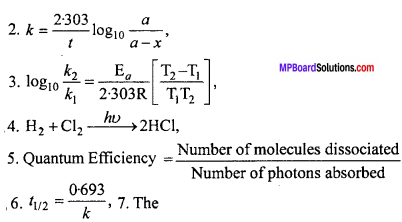7. The Number of photons absorbed minimum amount of energy which the reactant molecules should possess for effective collision.

Chemical Kinetics Very Short Answer Type Questions

Question 1.
What is the effect of rate constant on temperature? How can this effect of temperature be measured quantitatively? (NCERT)
Rate of reaction increases with increase in temperature and with 10°C rise in
temperature its value becomes two times. Arrhenius equation expresses the effect of temperature on constant
K = Ae-E /RT
Where A is frequency factor and Ea is activation energy of the reaction.Question 2.
The rate of reaction for A + 2B → Product, will be equal to it$$\frac {-d[A]}{dt}$$ = k[A] [B]2 if B is present in excess amount then what will be the order of reaction?
It will be 1st order of reaction.

Question 3.
Write alternative form of Arrhenius equation.Question 4.
For a zero order reaction t1/2is proportional to what?
Initial concentration of reactant [A].

Question 5.
What is Energy Barrier?
The minimum energy achieved by the reactant only after which it can be converted to product is known as energy barrier. Reactant molecules cannot form activated complex till they reach this height (activation energy) and cannot be converted to form product

Question 6.
Explain the rate determining step.
Some chemical reactions complete in one or more steps. Rate of reaction is determined by the slowest step which is known as rate determining step.

Question 7.
If unit of rate constant is litre/mole/sec. then what will be the order of reaction?
Order of reaction = Second.

Question 8.
Order of a reaction is zero can its molecularity be zero?
Molecularity of a reaction is always a whole number. Thus, it cannot be zero.Question 9.
What is specific reaction rate?
Specific reaction rate of a reaction at a given temperature is equal to that rate of a reaction when concentration of each reactant is unity.
Reaction : A2(g) + B2(g) → 2ABg
Rate of reaction ∝ [A2][B2]
If [A2] = [B2] = 1, then
Rate of reaction = k, where k = Specific reaction rate constant.

Question 10.
When is the average rate of the reaction equal to its instantaneous rate?
When value of time interval is nearly zero or when time by infinite form is minute then the average rate of reaction is comparable to its instantaneous rate.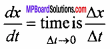Question 11.
What are pseudo unimolecular reactions?
There are some reactions in which molecularity is more than one i.e. two or more molecules are present, but in the chemical reaction concentration of only one reactant molecule is changed and it is only responsible for rate of reaction. Thus, order is one. These reactions are called pseudo unimolecular reactions.
Example: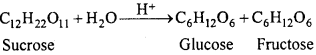Above reaction is bimolecular but concentration of water does not affect the rate of reaction, thus, rate of reaction is proportional to the concentration of sucrose only.
Rate = k[C12H22O11]
Thus, inversion of sucrose is a first order reaction. It is known as pseudo unimolecular reaction.

Question 12.
What is temperature coefficient?
Generally, rate of any reaction increases due to increase in temperature. On increasing temperature by 10°C, velocity of reaction is increased up to 2 – 3 times. If velocity constant of a reaction is and it is increased to increasing temperature by 10°C, the ratio of these two constant is called temperature coefficient, i.e.,
$$\frac { { k }_{ t+10 } }{ { k }_{ t } }$$ ≈ 2 to 3
Thus, at various time the ratio of rate of reaction which differ by 10°C is known as temperature coefficient.

Question 13.
As compared to water, gasoline vaporizes faster. Why?
Value of activation energy of vaporization of gasoline is less than the value of activation energy of water.

Chemical Kinetics Short Answer Type Questions

Question 1.
The conversion of molecules x to y follows second order kinetics. If concetration of x is increased to three times how will it affect the rate of formation of y? (NCERT)
Solution:
For the reaction x → y
Rate of reaction (r) = k[x]2 …. (1)
If concentration of x is increased three times, now
Rate of reaction (r)1 = k[3x]2 = k[9x2 ] …. (2)
Dividing equation (2) by Question (1),
$$\frac { { r }^{ 1 } }{ r }$$ = $$\frac { k[9{ x }^{ 2 }] }{ k[{ x }^{ 2 }] }$$ = 9
Thus, the rate of reactions will become 9 times.

Question 2.
Write down the expression representing the rate of reaction.
Rate of reaction is the rate of change in concentration of reactant or concentration of product in unit time interval.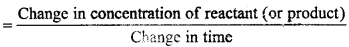Unit of rate of reaction depend on the units of concentration and time. If concentration is represented in mole per litre and time in second, then unit of rate of reaction is mol per litre per second. If time is expressed in minute then unit of reaction rate is mole per litre per minute.Question 3.
What is the meaning of instantaneous rate of reaction?
The rate of reaction does not remain constant during the whole time interval because rate of reaction depends upon the concentration of reactants. As the concentration of reactants decreases with time, the rate of reaction also decreases with time.
In order to express the reaction rate as accurately as possible, the instantaneous rate of reaction is expressed. For this the time interval (∆t) is taken as small as possible.
Instantaneous rate of reaction = –[$$\frac {∆A}{∆t}$$∆t → o
= – $$\frac {d[A]}{dt}$$
Where, d [A] is a change in concentration of reactant A.

Question 4.
What do you know about molecularity of any reaction?
Molecularity of Reaction:
‘Number of moles of reactant participating in elementary step of chemical reaction is called molecularity of the reaction.’
There are many reactions which proceed through the number of steps and each step being independent is called elementary step. The rate of reaction is determined by slowest step and it is known as rate determining step. Here molecularity can be defined as “Number of molecules/atoms or ions participating in rate determining step is called molecularity.”
Example:
(i) Unimolecular reaction :
O3 → O3 + O
(ii) Bimolecular reaction:
NO + O3 → NO2 + O2

Question 5.
Differentiate between molecularity and order of reaction. (MP 2018)
Differences between Molecularity and Order of reaction :
Molecularity:

• It is the total number of molecules which participate in the reaction.
• It is a theoretical concept.
• It is always a whole number.
• Its value is never zero.
• It does not provide any information about mechanism of reaction.

Order of reaction:

• It is the number of molecules which participate in reaction and whose concentration is changed.
• Order of reaction is determined by experimentally.
• Fractional values are also possible.
• Zero value is possible.
• It provides information about mechanisms of reaction.

Question 6.
Write any four factors which affects rate of a chemical reaction.
The rate of reaction depends upon the following factors:
1. Concentration of reactants:
At constant temperature, the rate of a reaction increases by increasing the concentration of the reactants.

2. Temperature of the system:
If the concentration of the reactants are constant then the rate of reaction increases by increasing temperature. For 10 degree rise of temperature, the reaction rate becomes double or triple.

3. Presence of catalyst:
Positive catalyst increases the rate of reaction and negative catalyst decreases the reaction rate.

4. Nature of the reactants:
Nature of reactants also affect the reaction rate. In any chemical reaction some old bonds are broken and new bonds are formed. Thus, in case of more simple molecules the lesser is the number of bond breaking and the rate of reaction increase whereas in case of complex molecules more bonds are broken and rate decreases.

The rate of some reactions increases due to some special radiations. For example: In the absence of light the reaction between hydrogen and chlorine is slow, but in the presence of light, the reaction proceeds at a faster rate.Question 7.
How does rate of any reaction depend on temperature? Explain.
Generally, rate of any reaction increases due to increase in temperature. On increasing temperature by 10°C, velocity of reaction is increased up to 2 – 3 times. If velocity constant of a reaction is and it is increased to increasing temperature by 10°C, the ratio of these two constant is called temperature coefficient, i.e.,
$$\frac { { k }_{ t+10 } }{ { k }_{ t } }$$ ≈ 2 to 3
Arrhenius provide the following relation for showing the effect of temperature on velocity constant.
k = A.e-Ea

Question 8.
Write a short note on activation energy?
According to Arrhenius, any chemical reaction is only possible when reacting molecules are activated with minimum energy which is called threshold energy. Kinetic energy of most of the molecules are less than this minimum energy. The excess energy which is required to activate reactant molecules, is called activation energy.
Activation energy can be determined by the use of Arrhenius equation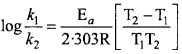Question 9.
Write four differences between Rate of reaction and Rate constant. (MP 2018)
Differences between Rate of reaction and Rate constant:
Rate of reaction:

• It is expressed in terms of consumption of reactants or formation of product per unit time.
• It depends on concentration of reactant at particular moment.
• It generally decreases with the progress of reaction.
• Its unit is mol L-1 cm-1.

Rate constant:

• It is proportionality constant in differential form in rate law or rate equation.
• It is independent of concentration of reactant.
• It does not depend on the progress of reaction.
• It changes according to order of reaction.

Question 10.
Prove that half – life period is independent of the initial concentration for the firsforder reaction.
Solution:
Half – life period for the reaction is that period in which the initial concentration of reactant is reduced to half.
For first order reaction :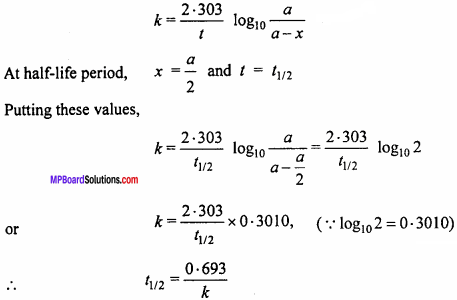Thus, the half – life period is independent of the initial concentration for the first order reaction.

Question 11.
(i) 2N2O5 → 4N02 +02
(ii) H2 +I2 → 2HI
Reaction (i) is first order reaction and (ii) is second order reaction. Why?
(i) In reaction 2N2O5 → 4NO2 + O2, when graph is plotted between rate of reaction and then again graph is plotted between rate of reaction and [N2O5]2, it is shown that in the first graph straight line is obtained i.e.
rate ∝ [N2O5]
or rate = k[N2O5]
Therefore, 2N2O5 → 4NO2 + O2 is first order reaction.

(ii) In reaction H2+I2 → 2HI when graph is plotted between rate of reaction and (H2) (I2), it is seen that straight line is obtained.
Therefore rate ∝ [H2][I2]
Hence, this reaction is of 2nd order reaction.Question 12.
What do you understand by order of reaction? Give example. (MP 2011,12,16)
Order of reaction:
The order of a reaction is defined as the sum of all the powers to which concentration terms in the rate law are raised to express the observecfrate of the reaction. Suppose there is a general reaction,
aA + bB + cC → product
For which the rate law is
Rate = – $$\frac {dx}{dt}$$ = k[A]p[B]q[C]r
Then the order of the reaction n = p + q + r, where, p, q and r are the orders with
respect to individual reactants and overall order is the sum of the exponents i.e.,p + q + r.
When n = 1 the reaction is of first order, if n = 2 the reaction is of second order and so on.
For example : Decomposition of ammonium nitrite occurs as follows :
NH4NO2 → N2 +2H2O
Rate of reaction = – $$\frac {dx}{dt}$$ = k [NH4NO2]
Thus, order of this reaction will be 1.

Question 13.
Write unit of rate constant k for the zero order, first order and second order reaction (MP 2012)Chemical Kinetics Long Answer Type Questions

Question 1.
Determine the expression for zero order reaction.
Reactions in which rate of reaction does not depend on the concentration of the rede tan ts are called zero order reactions. Consider a zero order reaction
A → B
Where, A and B are concentration of reactants and products. Since in this type of reaction, rate of reaction does not depend on the concentration of reactant therefore, rate of change in concentration of reactant remains constant.
Rate of reaction = Constant.
Let initial cone, of reactants be a moles /It and after time‘t’ x moles are converted into product. Then cone, of A after time t will be (a – x) mol /It.
$$\frac {-d[A]}{dt}$$ or $$\frac {dx}{dt}$$ ∝(a – x)0
or $$\frac {dx}{dt}$$ = k0(a – x)(a – x)0 … (1)
Where, k0 is velocity constant of a zero order reaction.
$$\frac {dx}{dt}$$ ∝ (a-x)(a – x)0 … (2)
dx = k0dt … (3)
Integrating equation. (2), we get
x = k0t + c … (4)
Where, c = integration constant
When, t = 0 then x = 0
Substituting it in equation. (4), we get
0 = k0 x O + C
or C = 0  …(5)
Substituting this in equation. (4), we get
x = k0t  … (6)
or k0 = $$\frac {x}{t}$$ = … (7)
Thus, equation. (7) is the velocity equation for zero order reaction. Unit of k
k0 = $$\frac {x}{t}$$
= $$\frac {mole/litre}{second}$$ = mole litre-1 second-1Theoretical yield calculator tool makes the calculation faster and it displays the theoretical yield value of the chemical reaction.

Question 2.
Write the Arrhenius equation in the form of equation of straight line. What will be the slope of a graph using this equation? Calculate the activation energy for the decomposition in a decomposition reaction in which the value of slope obtained is – 9920 when log k is plotted against $$\frac {1}{T}$$
Solution:
Arrhenius equation : Arrhenius gave a relation between rate constant of reaction and temperature which is known as Arrhenius equation, i.e.,
k = Ae-Ea/RT
Where, k = Rate constant of reaction, A = Frequency factor, Ea= Activation energy, R = Gas constant and T = Absolute temperature.Taking logarithm both sides of above equation, we get
loge k = – $$\frac { { E }_{ a } }{ RT }$$ + loge A
or log10 k = log10 A – $$\frac { { E }_{ a } }{ 2.303RT }$$ .. (i)
This is a straight line equation. If log10 k and $$\frac {1}{T}$$ is plotted at different temperatures, a straight line is obtained whose slope = $$\frac { { -E }_{ a } }{ 2.303RT }$$ From the graph, value of slope = $$\frac { { E }_{ a } }{ 2.303RT }$$ can be calculated and activation energy (Ea ) can be calculated.
Slope = $$\frac { { -E }_{ a } }{ 2.303RT }$$ (Activation energy)
Or Ea = Slope x 2.303 x R
Or Ea = – 9920 x 2.303 x (-4.58)
∴ Ea = 104633.5808 calorie per gm moleculeQuestion 3.
Derive an expression for rate constant of first order reaction.
The reaction in which rate of reaction depends upon the concentration of one mole, are called first order reaction.
Let this reaction is
A → product
Suppose intial concentration of A is a gram mole and after t second x mole consumed and remaining concentration is (a – x) gram mole.
So after t time, the rate of reaction will be proportional to (a – x)
$$\frac {dx}{dt}$$ ∝(a – x)
or $$\frac {dx}{dt}$$ = k (a – x) (k = Velocity constant) … (1)
or $$\frac {dx}{(a – x)}$$ = kdt … (2)
on intergating this equation,
∫$$\frac {dx}{(a – x)}$$ = ∫ kdt
or -ln(a – x) = kt + c
Where c is intergating constant, …. (3)
If t = 0 then x = 0
Putting the value of c from eqn. (3),
-ln a = c .. (4)
Putting the value of c from eqn. (4), into eqn. (3).
-ln(a – x) = kt – ln a
or ln a – ln (a – x) = kt
ln $$\frac {a}{(a – x)}$$ = kt
or k = $$\frac {1}{(t)}$$ln$$\frac {a}{(a – x)}$$
charging the base of log,
k = $$\frac {2.303}{(t)}$$ log $$\frac {a}{(a – x)}$$
It is the desired expression for first order of reaction

Chemical Kinetics Numerical Questions

Question 1.
A first order reaction has a rate constant 1.15 x 10-3 s-1 How long will 5g of this reactant take to reduce to 3g? (NCERT)
Solution:
According to question,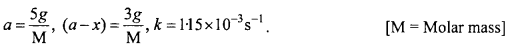Applying first order kinetic equationQuestion 2.
The rate of the chemical reaction doubles for an increase of 10K in absolute temperature from 298K. Calculate Ea.
Solution:Question 3.
The reaction between A and B is first order with respect to A and zero order with respect to B. Fill in the blanks in the following table: (NCERT)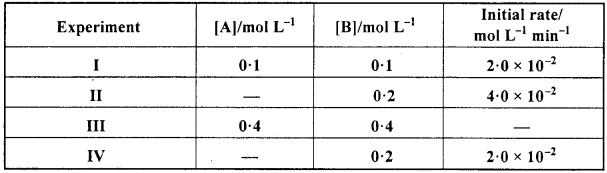Solution:
Given that the reaction between A and B is first order w.r.t. A and zero order w.r.t. B.
Rate = k [A]1[B]0 but [B]0 = I.
Rate = k [A]
From experiment I,
2 x 10-2 = k (0. 1) ⇒ k = 0.2 min-1
From experiment II,
4 x 10-2 = 0.2 [A] ⇒[A] = 0.2 min L-1
From experiment III,
Rate = (0.2) (0.4) = 0 08 mol L-1 min-1
From experiment IV,
2 x 10-2= 0.2 [A] ⇒ [A] = 0.1 mol L-1.Question 4.
For a first order reaction, show that time required for 99% completion is twice the time required for the completion of 90% of reaction. (NCERT)
Solution: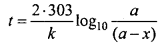If 90% reaction is getting completedIf 99% reaction is getting completedDividing equation (1) by equation. (2),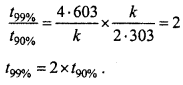Question 5.
A first order reaction takes 40 min for 30% decomposition. Calculate tm. [CBSE (Delhi) 2013], (NCERT)
Solution:
30% decomposition means that x = 30% of a = 0.30 a
As reaction is of first order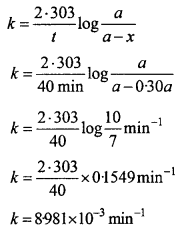For a first order reaction,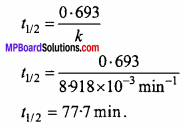Question 6.
Show that time required for completing 99.9% of a first order reaction is 10 times of its half life period.
Solution:
If initial concentration of reactant is a, then t = ? for x = 0.999 a
We know that for a first order reactionMP Board Class 12th Chemistry Important Questions